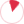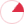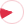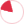## Schedule for today

 D.V. BolotovV.O. GorkavyyE.V. PetrovO.O. ShugailoWeek schedule# Alexander L. Yampolsky

Docent (associate professor) of department of fundamental mathematics, z, doctor of sciences in physics and mathematics, docent (associate professor)

## List of selected publications

A. Yampolsky On stability of left invariant totally geodesic unit vector fields on three dimensional Lie group // Geometry and its Applications , Springer Proceedings in Mathematics & Statistics, Rovenski, Vladimir, Walczak, Paweł (Eds.) , 2014, Vol. 72 , p. 167 - 195, 2013

We consider the problem on stability or instability of unit vector fields on three-dimensional Lie groups with left-invariant metric which have totally geodesic image in the unit tangent bundle with the Sasaki metric with respect to classical variations of volume. We prove that among non-flat groups only SO(3) of constant curvature +1 admits stable totally geodesic submanifolds of this kind. Restricting the variations to left-invariant (i.e., equidistant) ones, we give a complete list of groups which admit stable/unstable unit vector fields with totally geodesic image.

Keywords: Sasaki metric, Lie group, stable submanifold

Yampolsky A. On geodesics of tangent bundle with fiberwise deformed Sasaki metric over Kahlerian manifold. // Journal of Math. Phys., Analysis, Geom., v. 8/2, p. 177-189, 2012

We propose a fiber-wise deformation of the Sasaki metric on slashed and unit tangent bundles over the Kalerian manifold based on the Berger deformation of metric on a unit sphere. The geodesics of this metric have dierent projections on a base manifold for the slashed and unit tangent bundles in contrast to usual Sasaki metric. Nevertheless, the projections of geodesics of the unit tangent bundle over the locally symmetric Kahlerian manifold still preserve the property to have all geodesic curvatures constant.

Keywords: Sasaki metric, Kahlerian manifold, tangent bundle, geodesics.

Yampolsky A. Minimal and totally geodesic sections of the unit sphere bundles. // Visnyk KhNU, ser. Math. App. Math and Mech. , v. 1030, p. 54 – 70, 2012

We consider a real vector bundle $E$ of rank $p$ and a unit sphere bundle $E_1 \subset E$ over the Riemannian $M^n$ with the Sasaki-type metric. A unit section of $E_1$ gives rise to a submanifold in $E_1$. We give some examples of local minimal unit sections and present a complete description of local totally geodesic unit sections of $E_1$ in the simplest non-trivial case $p = 2$ and $n = 2$.

Totally geodesic vector fields on pseudo-Riemannian manifolds. // Visnyk Kharkiv Karazin Univ., ser Math, App. Math and Mech, , v. 990, p. 4 - 14, 2011

We consider the submanifolds in the unit tangent bundle of the pseudo- Riemannian manifold generated by the unit vector ﬁelds on the base. We have found the second fundamental form of this type of submanifolds with respect to the normal vector ﬁeld of a special kind. We have derived the equations on totally geodesic non-isotropic unit vector ﬁeld. We have found all the two-dimensional pseudo-Riemannian manifolds which admit non- isotropic totally geodesic unit vector ﬁelds as well as the ﬁelds.

Invariant totally geodesic unit vector fields on three-dimensional Lie groups // Journal of Mathematical Physics, Analysis, Geometry, vol. 3, No. 2, pp. 253 - 276, 2007

We give a complete list of left-invariant unit vector elds on three- dimensional Lie groups equipped with a left-invariant metric that generate a totally geodesic submanifold in the unit tangent bundle of a group equipped with the Sasaki metric. As a result we obtain that each three-dimensional Lie group admits totally geodesic unit vector eld under some conditions on structural constants. From a geometrical viewpoint, the eld is either parallel or a characteristic vector eld of a natural almost contact structure on the group.

Keywords: Sasaki metric, totally geodesic unit vector eld, almost contact structure, Sasakian structure.

Yampolsky A. // Dokl. Ukr. Acad Nauk, v.3, p. 32-35, 2005

Totally geodesic submanifolds in the tangent bundle of a Riemannian 2-manifold. // Journal of Mathematical Physics, Analysis, Geometry, v.1/1, p. 116-139, 2005

We give a full description of totally geodesic submanifolds in the tangent bundle of a Riemannian 2-manifold of constant curvature and present a new class of a cylinder-type totally geodesic submanifolds in the general case.

On special types of minimal and totally geodesic unit vector fields. // 7-th International Conference on Geometry, Integrability and Quantization, June 2-10, Varna (Bulgaria), SOFTEX, Sofia, p. 290 – 304, 2005

We present a new equation with respect to a unit vector ﬁeld on Riemannian manifold $M^n$ such that its solution deﬁnes a totally geodesic submanifold in the unit tangent bundle with Sasaki metric and apply it to someclasses of unit vector ﬁelds. We introduce a class of covariantly normal unit vector ﬁelds and prove that within this class the Hopf vector ﬁeld is a unique global one with totally geodesic property. For the wider class of geodesic unit vector ﬁelds on a sphere we give a new necessary and sufﬁcient conditionto generatea totally geodesic submanifold in $T_1S^n$.

Keywords: Sasaki metric, minimal unit vector ﬁeld, totally geodesic unit vector ﬁeld, strongly normal unitvector ﬁeld,Sasakian space form.

Abbassi M.T.K., Yampolsky A. // Math. Publ. Debrecen, v.64 /1-2, p. 129-154, 2004

Yampolsky A., Saharova E. // Ukr. Math. Journal, v.56/9, p. 1231-1243, 2004

A. Yampolsky Full description of totally geodesic unit vector field on Riemannian 2-manifold. // Matematicheskaya fizika, analiz, geometriya, 2004, v.11/3, p.355-365, 2004

We give a full geometrical description of local totally geodesic unit vector field on Riemannian 2-manifold, considering the field as a local embedding of the manifold into its unit tangent bundle with the Sasaki metric.

Keywords: Sasaki metric, totally geodesic unit vrctor field

A. Yampolsky Totally geodesic property of the Hopf vector field. // Acta Math. Hungarica, 2003, v.101, № 1-2, p. 73-92, 2003

We prove that the Hopf vector field is a unique one among geodesic unit vector fields on spheres such that the submanifold generated by the field is totally geodesic in the unit tangent bundle with Sasaki metric. As application, we give a new proof of stability (instability) of the Hopf vector field with respect to volume variation using standard approach from the theory of submanifolds and find exact boundaries for the sectional curvature of the Hopf vector field.

Keywords: Sasaki metric, Hopf vector field, curvature

A. Yampolsky On extrinsic geometry of unit normal vector field of Riemannian hyperfoliation. // Math. Publ. Debrecen, v.63/4, p. 555-567, 2003

We consider a unit normal vector feld of (local) hyperfoliation on a given Riemannian manifold as a submanifold in the unit tangent bundle with Sasaki metric. We give an explicit expression of the second fundamental form for this submanifold and a rather simple condition its totally geodesic property in the case of a totally umbilic hyperfoliation. A corresponding example shows the non-triviality of this condition. In the 2-dimensional case, we give a complete description of Riemannian manifolds admitting a geodesic unit vector feld with totally geodesic property.

Keywords: Sasaki metric, hyperfoliation

A. Yampolsky On the mean curvature of a unit vector field. // Math. Publ. Debrecen, v.60, 1/2, p. 131-155, 2002

We present an explicit formula for the mean curvature of a unit vector field on a Riemannian manifold, using a special but natural frame. As applications, we treat some known and new examples of minimal unit vector fields. We also give an example of a vector field of constant mean curvature on the Lobachevsky (n + 1) space.

Keywords: Sasaki metric, minimal unit vector field

A. Yampolsky On the intrinsic geometry of a unit vector field. // Comment. Math. Univ. Carolinae 2002,, v.43, № 2, p. 299-317, 2002

We study the geometrical properties of a unit vector field on a Riemann- ian 2-manifold, considering the field as a local imbedding of the manifold into its tangent sphere bundle with the Sasaki metric. For the case of con- stant curvature K, we give a description of the totally geodesic unit vector fields for K = 0 and K = 1 and prove a non-existence result for K= 0 and K=1. We also found a family of vector fields on the hyperbolic 2-plane L^2 of curvature -c^2 which generate foliations on T_1L^2 with leaves of constant intrinsic curvature -c^2 and of constant extrinsic curvature -c^2/4 .

Keywords: Sasaki metric, totally geodesic submanifold

Yampolsky A. // Math. phys., analysis and geometry, v.3, No ¾, p. 446-456, 1996

Yampolsky A. // Math. phys., analysis and geometry, v.1, No 3/4, pp. 540-545, 1994

Yampolsky A. // Ukr. geom. sbornik, v.35, pp. 150-159, 1992

Yampolsky A. // Ukr. geom. sbornik, v.34, pp. 121-126, 1991

Yampolsky A., Borisenko A.A. // Uspehi math. nauk, No 6, pp 51-95, 1991

Yampolsky A. // Ukr. geom. sbornik, v.32, pp. 127-137, 1989

Yampolsky A.., Borisenko A.A. // Ukr. geom. sbornik, v.30 , pp.10-17, 1987

Yampolsky A., Borisenko A.A. // Dokl. Acad. Sci. USSR, v. 294, No 1, pp. 19-22, 1987

Yampolsky A., Borisenko A.A. // Math. Sbornik, v.134, No 2, pp. 158-176, 1987

Yampolsky A.., Borisenko A.A. // Ukr. geom. sbornik, v.29, p. 12-32, 1986

Yampolsky A. // Ukr. geom. sbornik, v.28, p. 132-145, 1985

Yampolsky A. To the geometry of tangent sphere bundle of the Riemannian manifold. // Ukr. geom. sbornik, v.24, p.129-132, 1981

The tangent sphere bundle $T_rM^2$ of Riemannian manifold M of dimension 2 was considered. The main result is: The sectional curvature of Sasaki metric of $T_rM^2$ is positive if and only if $|grad K|^2 < K^3(1-3/4r^2 K)$.

Keywords: Sasaki metric, sectional curvature

//

A. Yampolsky A, O.Fursenko Caustics of wave fronts reflected by a surface // Journal of Mathematical Sciences and Modeling, 2018, V 1 , Issue 2, Pages 131 - 137. https://doi.org/10.33187/jmsm.431543,

A. Yampolsky, A. Opariy Generalized helices in three-dimensional Lie groups // , Turkish Journal of Mathematics (2019) , 43: 1447 – 1455. http://journals.tubitak.gov.tr/math/,

A. Yampolsky Catacaustics of a hypersurface in the Euclidean n-space. // Mediterranean Journal of Mathematics, , (2019) 16: 88. https://doi.org/10.1007/s00009-019-1365-3,

A. Yampolsky On Projective Classication of Points of a Submanifold in the Euclidean Space. // Journal of Mathematical Physics, Analysis, Geometry., 2020. V. 16, № 3, P. 364–371. ,

A. Yampolsky On properties of the Reeb vector field of (α,β) trans-Sasakian structure. // Turkish Journal of Mathematics, 2022,, Vol. 46: No. 6, Article 19. ,